# Introduction To Ellipses

Go back to  'Ellipse'

Parabolas, which we studied in the last chapter, are conic sections with eccentricity $$e = 1.$$ In this section, we study ellipses, which have $$e < 1.$$ This means, by definition, that an ellipse is the locus of a moving point such that the ratio of its distance from a fixed point to its distance from a fixed line is a constant less than unity. In fact we will soon discover that an ellipse has two foci and two directrices.

We now start by first writing down the basic equations representing an ellipse.

Let $$F$$ represent the focus of an ellipse and $$L$$ be its directrix. Let $$Q$$ be any point on the ellipse.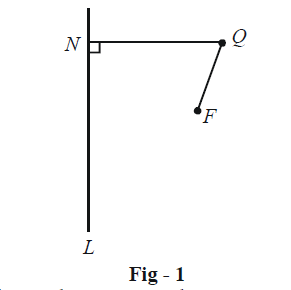If the eccentricity of this ellipse is given to be $$e,$$ we must have

$\frac{{\left( {{\rm{Distance \;of\; }}Q{\rm{\; from \;}}F} \right)}}{{\left( {{\rm{Distance \;of\; }}Q{\rm{ \;from\; the\; line\; }}L} \right)}} = \frac{{QF}}{{QN}} = e$

Draw a line through $$F$$ perpendicular to the directrix $$L,$$ which meets the directrix at $$M.$$ On this line, we can always find two points, say $$P$$ and $$P',$$ which divide the line segment $$MF$$ internally and externally respectively in the ratio $$1:e.$$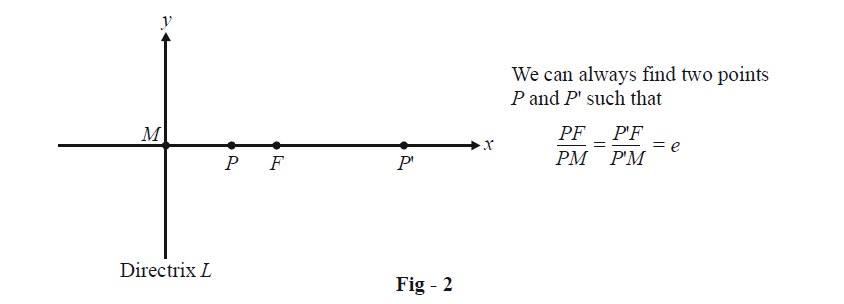Thus, by definition, $$P$$ and $$P'$$ will both lie on the ellipse.

We will now introduce co-ordinates into this geometry. We assume the $$x\text{-axis}$$ to coincide with the horizontal line and the origin to be at the mid-point of $$PP'.$$ The co-ordinates of $$P$$ and $$P'$$ can be assumed to be $$(–a, 0)\text{ and }(a, 0):$$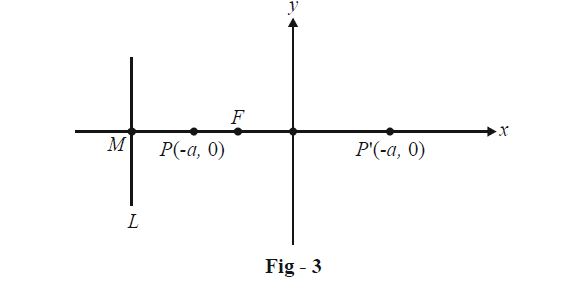Since \begin{align}\frac{{PF}}{{PM}} = e \text{ and }\frac{{P'F}}{{P'M}} = e, \end{align} and we have

\begin{align}&PF + P'F = e(PM + P'M)\\\Rightarrow \qquad & PP' = e(PM + P'M)\\\Rightarrow\qquad &2a = e(2PM + PP')\\&{\rm{ }}\quad= 2ePM + 2ae\\\Rightarrow \qquad & PM = \frac{a}{e}(1 - e)\end{align}

This gives \begin{align}PF = ePM = a(1 - e) \text{ and }OM = OP + PM = \frac{a}{e}.\end{align} Thus the co-ordinates of $$F$$ are $$(–ae, 0)$$ and that of $$M$$ are \begin{align}\left( { - \frac{a}{e},\,\,0} \right).\end{align}

Till now we have assumed $$F$$ to be the focus of the ellipse and $$L$$ to be the directrix. However, by the symmetry inherent in Fig -3, you might be able to infer that there should exist another focus $$F'$$ and directrix $$L ',$$ to the right of $$O$$ as shown below: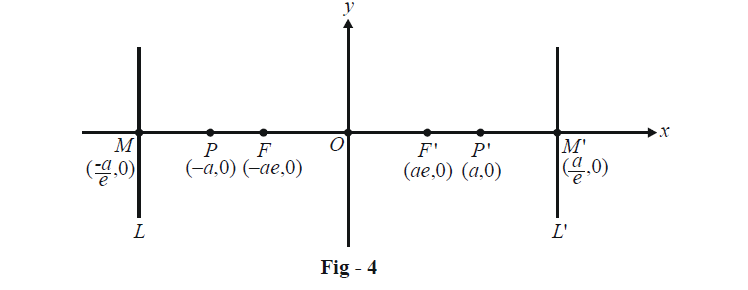That there exist another focus and directrix will become evident once we obtain the equation of the ellipse.

To find the equation of the ellipse we assume the co-ordinates of any point $$Q$$ lying on the ellipse as $$(x, y):$$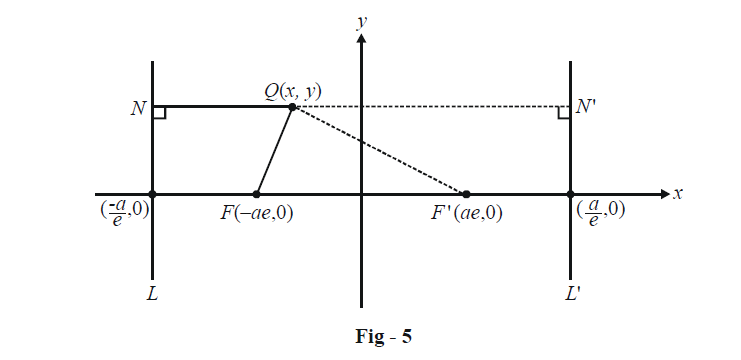We thus have

\begin{align}&\qquad\frac{{QF}}{{QN}} = e \hfill \\&\Rightarrow\quad \frac{{{{(x + ae)}^2} + {y^2}}}{{{{\left( {x + \frac{a}{e}} \right)}^2}}} = {e^2} \hfill \\&\Rightarrow \quad {x^2} + {y^2} + {a^2}{e^2} + 2aex = {e^2}{x^2} + {a^2} + 2aex \hfill \\&\Rightarrow\quad {x^2}(1 - {e^2}) + {y^2} = {a^2}(1 - {e^2}) \hfill \\&\Rightarrow\quad \frac{{{x^2}}}{{{a^2}}} + \frac{{{y^2}}}{{{a^2}(1 - {e^2})}} = 1 \hfill \\&\Rightarrow\quad \boxed{\frac{{{x^2}}}{{{a^2}}} + \frac{{{y^2}}}{{{b^2}}} = 1}\qquad\qquad{\text{ }} where{\text{}}\;{b^2} = {a^2}(1 - {e^2}) \hfill \\\end{align}

This is the equation of the ellipse; any point $$(x, y)$$ on the ellipse must satisfy this equation.

The symmetric nature of this equation should convince you that there does indeed exist another focus $$F'$$ and another directrix $$L '.$$ In fact, you are now urged to verify the truth of this explicitly by proving that for any point $$Q (x, y)$$ lying on the ellipse as in Fig - 5, we always have

$\frac{{QF}}{{QN}} = \frac{{QF'}}{{QN'}} = e$

We finally plot the ellipse taking help of the equation obtained.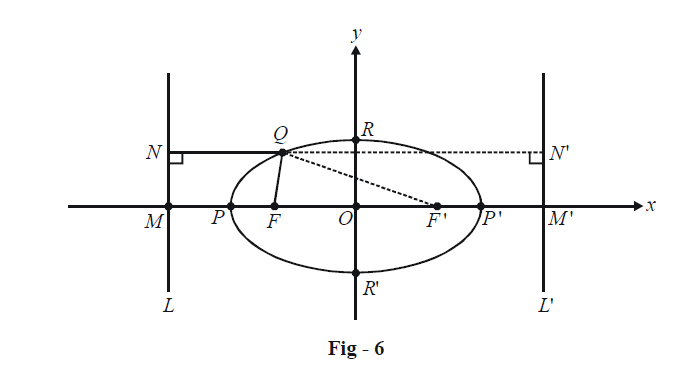The co-ordinates of $$R$$ and $$R'$$ can be obtain by substituting $$x = 0$$ in the equation of the ellipse obtained:

$y = \pm b$

Thus, $$R \equiv (0,\,b)$$ and $$R' \equiv (0,\, - b)$$.

$$PP',$$ whose length equals $$2a,$$ is termed the major-axis of the ellipse, while $$RR ',$$ whose length equals $$2b,$$ is termed the minor-axis of the ellipse. The names are self-explanatory. The coordinates of $$F$$ and $$F'$$ have already been mentioned: $$(–ae, 0)$$ and $$(ae, 0)$$ so that $$FF' = 2ae.$$ Also, \begin{align}MM' = \frac{{2a}}{e}.\end{align}

We now note a very important fact about any ellipse from Fig - 6. Since $$Q$$ lies on the ellipse, we have by definition,

\begin{align}&\quad\quad\;\frac{{QF}}{{QN}} = \frac{{QF'}}{{QN'}} = e\\&\Rightarrow\qquad QF + QF' = e(QN + QN')\\&\qquad\qquad\qquad\qquad= e(NN')\\&\qquad\qquad\qquad\qquad= e(MM')\\&\qquad\qquad\qquad\qquad= 2a\end{align}

This means that for any point on the ellipse, the sum of its distances from the two foci is a constant, and equal to the major axis of the ellipse. In fact, this property itself is sometimes used to define an ellipse:

$\boxed {\rm{\text{An ellipse is a locus of a moving point }}Q{\text{ such that the sum of its distances from two fixed }} \\{\text{points }}F{\text{ and }}F'{\text{, i}}{\text{.e}}{\text{., }}QF{\text{ + }}QF'{\text{, is a constant}}{\text{. }}F{\text{ and }}F'{\text{ are termed the foci of the ellipse}} }$

The two definitions of the ellipse, one through ‘eccentricity’ and one through the ‘constant sum of distances’, are equivalent.

There is a very nice way to plot an ellipse very precisely. Fix two pegs on a board at a distance l, and tie a string of length $$L > l$$ between these two pegs. Using your pen, stretch this string away from the pegs, so that it becomes taut: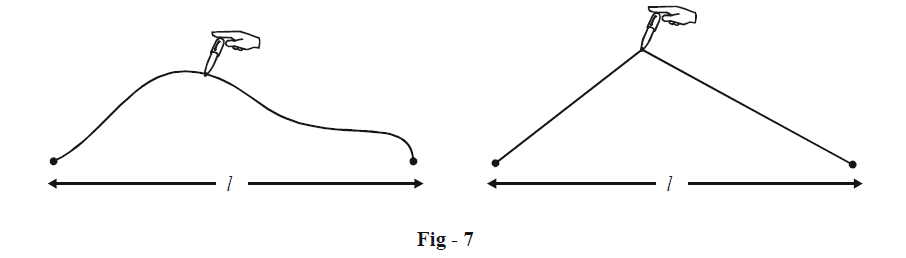Now always keeping the string taut, mark out a complete revolution on the paper with the pen. You’ve just made an ellipse! This is because keeping the string taut ensures that the sum of the distances of the tip of your pen from the two foci is always constant, equal to $$L.$$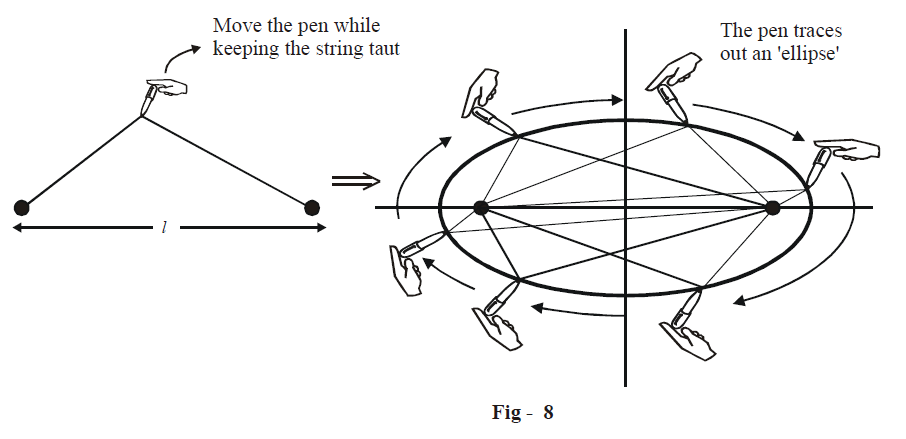Can you calculate the eccentricity of this ellipse we just made? If we compare this ellipse with the standard form obtained earlier, we have

\begin{align}&2ae = l\\&\;\,2a = L\\\Rightarrow\qquad & \;\;\;\, e = \frac{l}{L}\end{align}